IF YOU CAN SEE THIS go to /sfa/Nuprl/Shared/Xindentation_hack_doc.html

### Recursion via the Y-combinator - from Recursive Functions

Consider the definition of factorial:

Def x! == if x=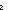0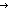1 else x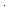(x-1)! fi  (recursive)

Observe that the operator "n!" occurs on both sides of the definition, connoting a recursive definition. There is no need for further explanation for the typical user's needs, but this form of operator definition is not built into the system, and here we explain how such definitions are implemented on top of the real primitives of Nuprl.

The computational basis of Nuprl's semantics is an untyped calculus, which includes the "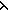x.b(x)" and "f(a)" operators.
Thus, we can build the computational fixed-point "combinator" Y:

Def Y(f) == (x.f(x(x)))(x.f(x(x)))

Now, observe how (x.f(x(x)))(x.f(x(x))) computes:

(x.f(x(x)))(x.f(x(x))) *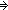f((x.f(x(x)))(x.f(x(x))))

One step of computation stuffs the whole original term into the argument place of an application of f; thus, whatever f is, (x.f(x(x)))(x.f(x(x))) is a fixed-point of evaluation. If we abstract from the variable f we getf.(x.f(x(x)))(x.f(x(x))) which can then be applied to various functions we want to take fixed-points of. This function abstraction is abbreviated as "Y":

Y ==f.(x.f(x(x)))(x.f(x(x)))

Normally, we display such definitions using applications to the variable on the lefthand side instead of using the abstraction operator on the right hand side, which comports better with standard mathematical practice: Def Y(f) == (x.f(x(x)))(x.f(x(x))).

With this definition in place, we can rewrite Y(f) thus:

Y(f) *(x.f(x(x)))(x.f(x(x))) *f((x.f(x(x)))(x.f(x(x)))) *f(Y(f))

Eliding intermediate steps pointedly demonstrates the fixed-pointedness Y(f) *f(Y(f)).

(Feb 2001 - sfa )